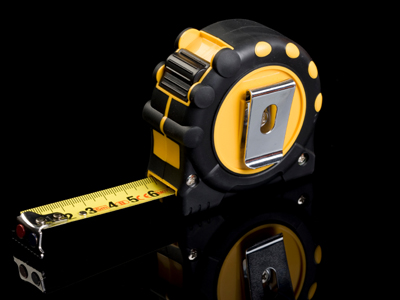Improve your knowledge of maths in this quiz.

# Upper & Lower Bounds (H)

You should be familiar with the idea of ROUNDING a number, either to a given number of SIGNIFICANT FIGURES (sf) or DECIMAL PLACES (dp). Working out ERROR BOUNDS is the opposite process – it is finding how big (UPPER BOUND) or how small (LOWER BOUND) the number could have been, before it was rounded. See how well you know your error bounds in this GCSE Maths quiz.

Error bounds are tied up with ACCURACY – the degree (usually given or implied) of how correct a number is. When working out error bounds, you need to go to the next level of accuracy. A few examples will help this make sense.

The height of a door is stated as 2m. This implies that the number is stated, to the nearest metre. How tall, and how small, COULD the door have been? It could have been as tall as 2.5m (but not including 2.5), as any number in this interval would round down to 2. Similarly, it could have been as small as 1.5m, as anything above this would round to 2m. We could write this as an inequality, 1.5m ≤ door height < 2.5m.

However, if the height is given as 2.00m then it is much more accurate, as it is given to the nearest hundredth of a metre (or nearest cm). The maximum height it could have been is now only 2.005m, or as small as 1.995m. This can be written as 1.995m ≤ door height < 2.005m.

With a bit of practise you should start to see the technique – add and subtract half a unit at the level of accuracy stated.

Calculating with error bounds needs a bit more care and planning, particularly when dividing is involved.

Question 1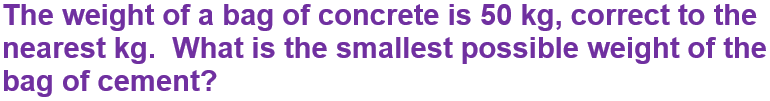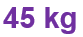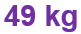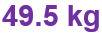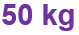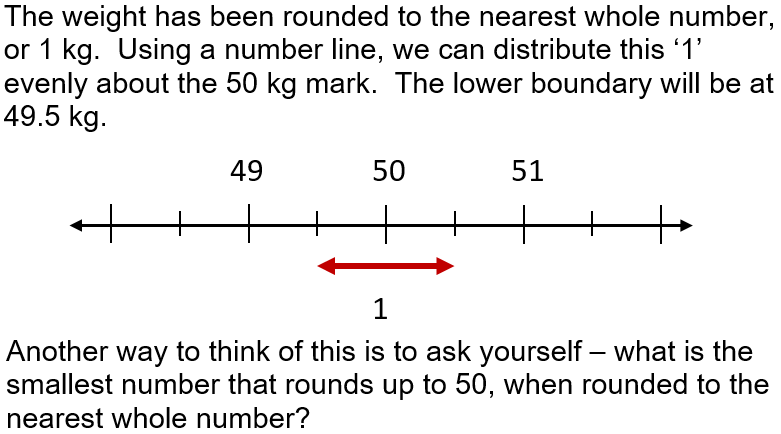Question 2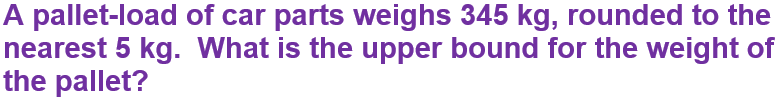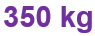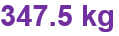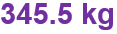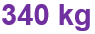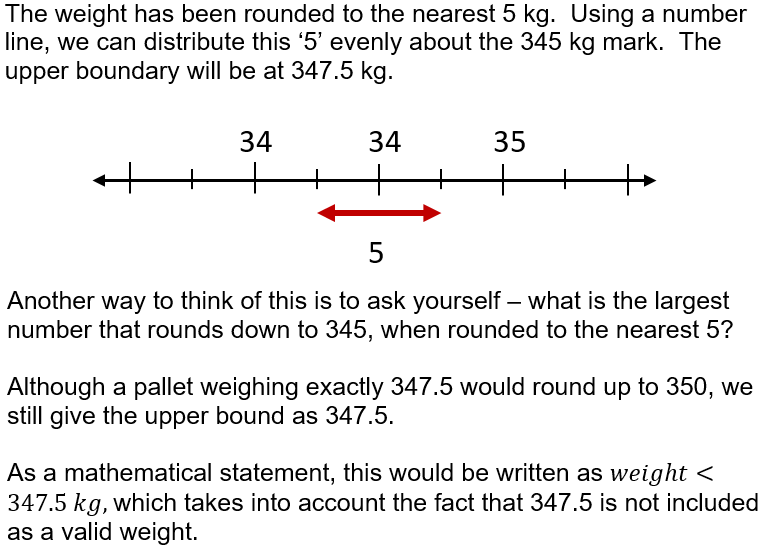Question 3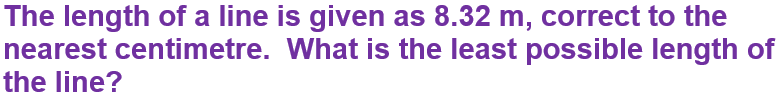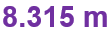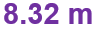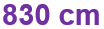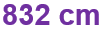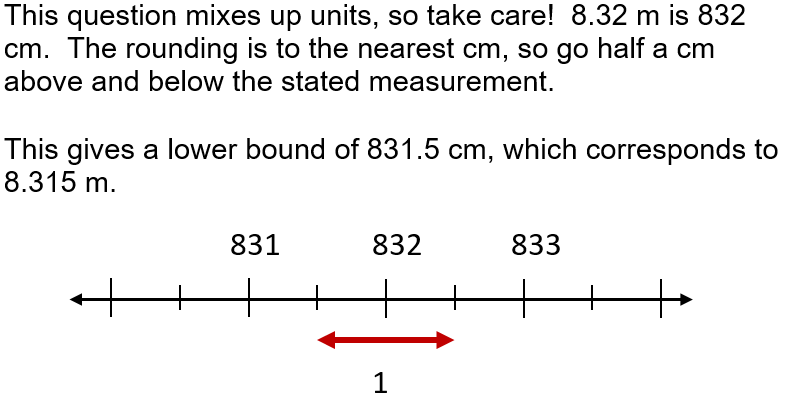Question 4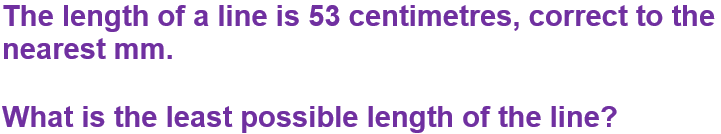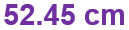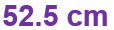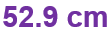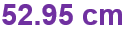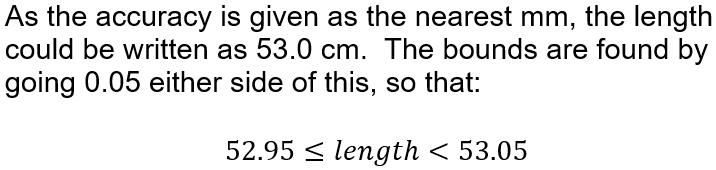Question 5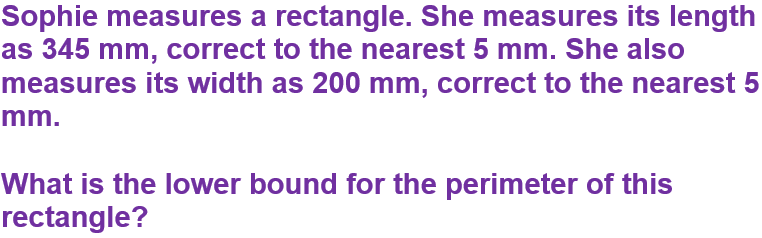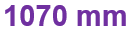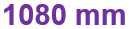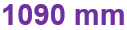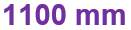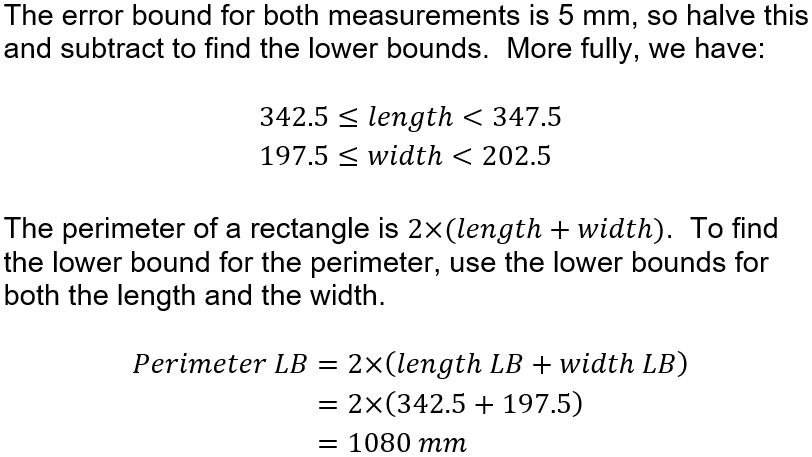Question 6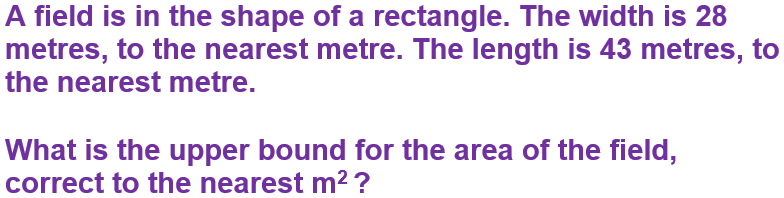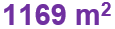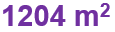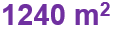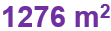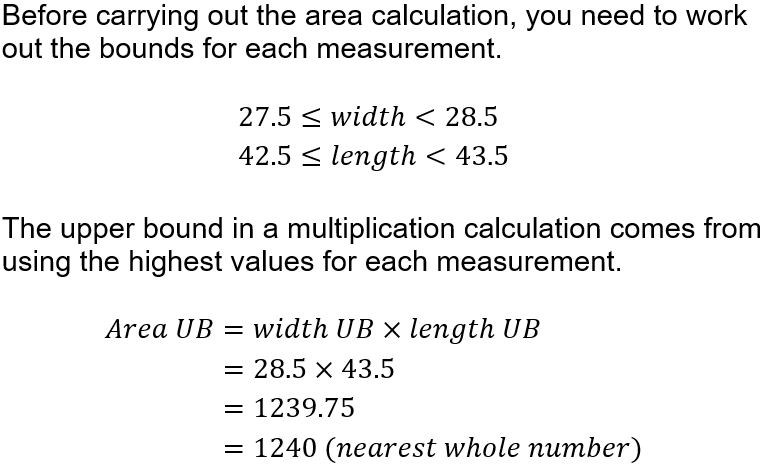Question 7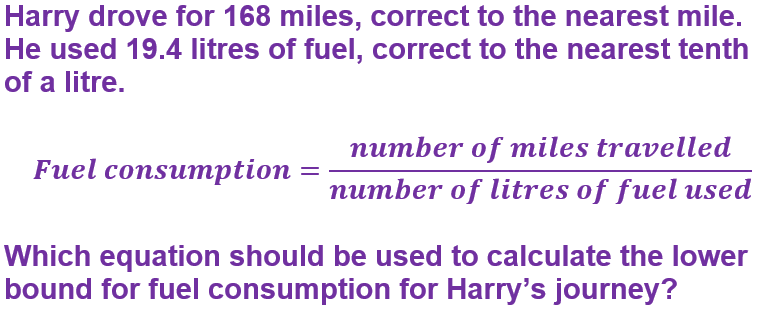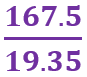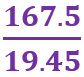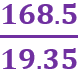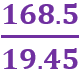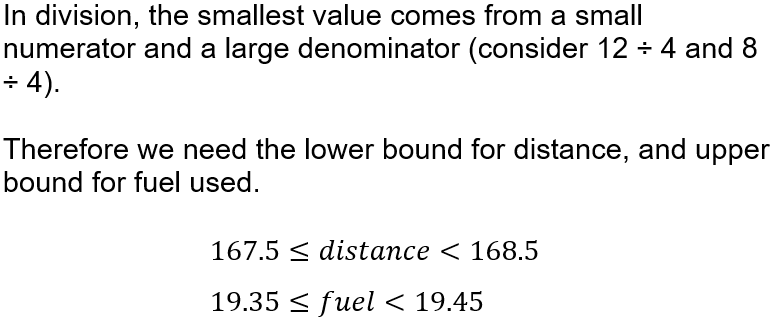Question 8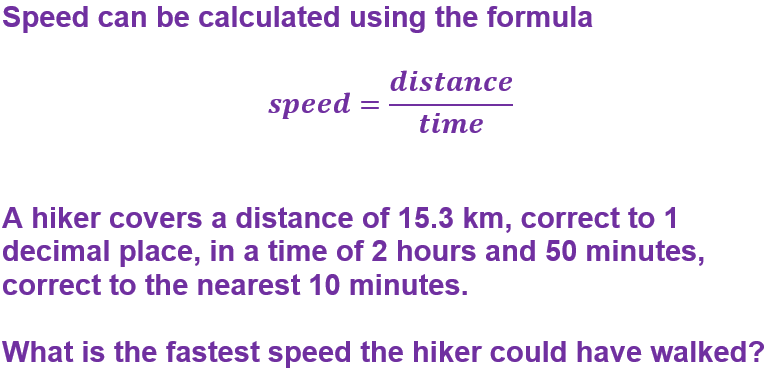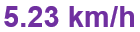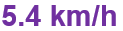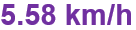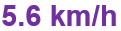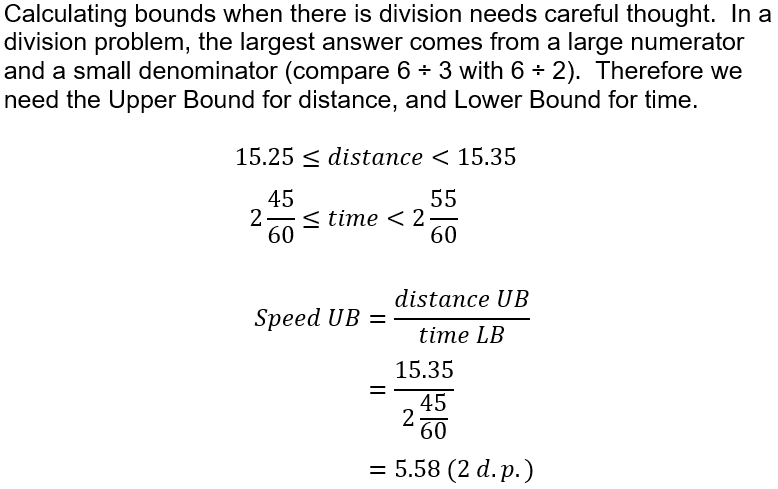Question 9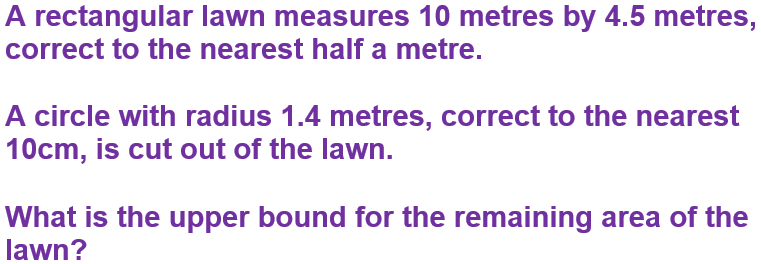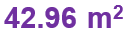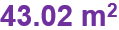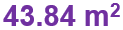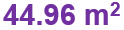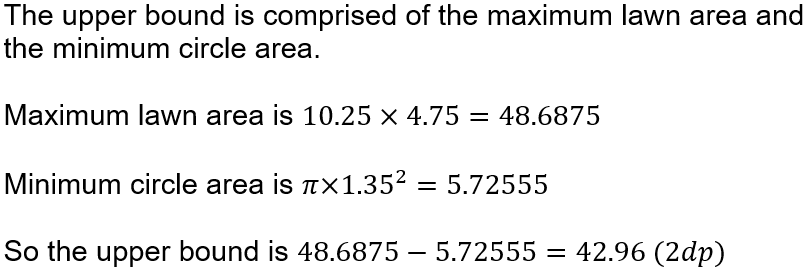Question 10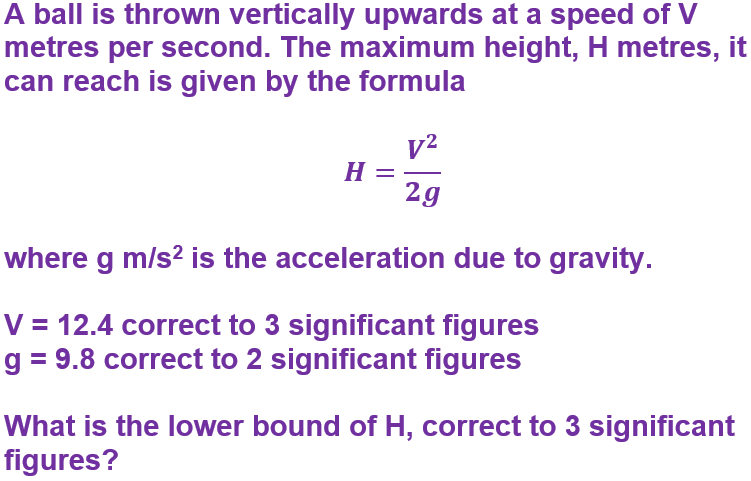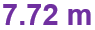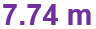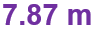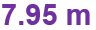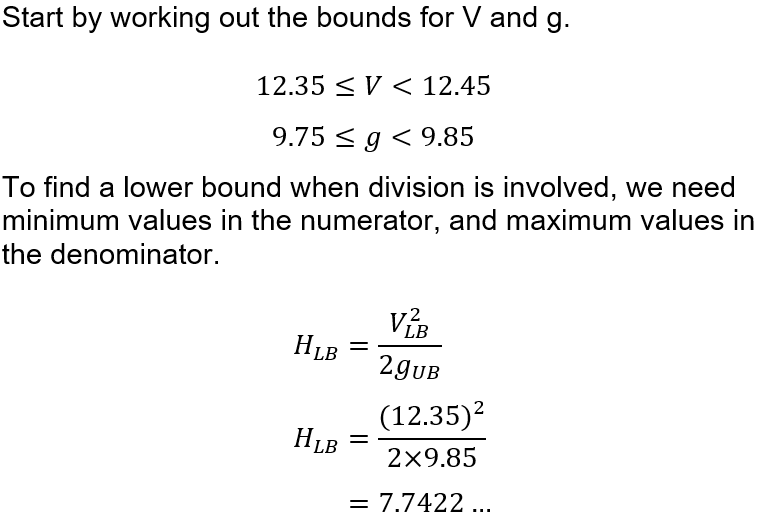Author:  Sally Thompson

We use cookies to make your experience of our website better.

To comply with the new e-Privacy directive, we need to ask for your consent -